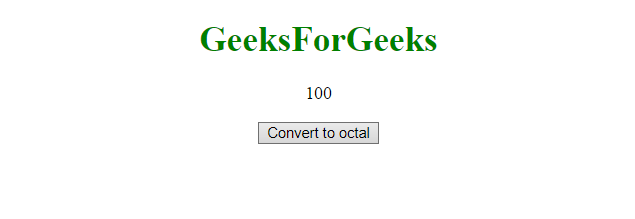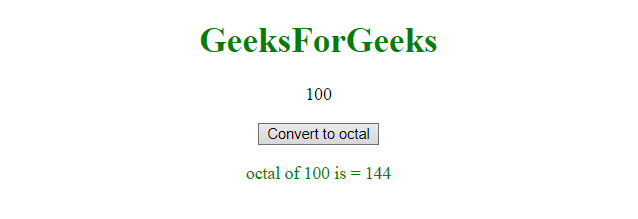# How to convert decimal to hex in JavaScript ?

Given a number and the task is to convert the number from decimal to hex. This can be done by using toString() method. It takes the parameter which is the base of the converted string. In this case the base will be 16.

Syntax:

`decimalNumber.toString( radix )`

Parameters: It accepts two parameters which are listed below:

• decimalNumber: It holds the number in decimal format which need to be converted.
• base: It holds the base of number system in which number is converted.

Example 1: This example converts 20 to hex number system.

 `  ` `<``html``>  ` ` `  `<``head``>  ` `    ``<``title``>  ` `        ``How to convert decimal to ` `        ``hex in JavaScript ? ` `    `` ` `  ` `         `  `<``body` `style` `= ``"text-align:center;"``>  ` `     `  `    ``<``h1` `style` `= ``"color:green;"` `>  ` `        ``GeeksForGeeks  ` `    ``  ` `             `  `    ``<``p` `id``=``"up"``> ` `     `  `    ``<``button` `onclick``=``"myGFG()"``>  ` `        ``Convert to hex ` `    ``  ` `     `  `    ``<``p` `id``=``"down"` `style``=``"color: green"``> ` `             `  `    ``<``script``>  ` `        ``var GFG_Var = 20; ` `        ``var up = document.getElementById("up"); ` `        ``up.innerHTML = GFG_Var; ` `        ``var down = document.getElementById("down"); ` `     `  `        ``function myGFG() { ` `            ``var GFG_Var2 = GFG_Var.toString(16);  ` `            ``down = document.getElementById("down"); ` `            ``down.innerHTML = "hex of "+ GFG_Var ` `                    ``+ " is = " + GFG_Var2; ` `        ``} ` `    ``  ` `  ` ` `  `                                 `

Output:

• Before clicking the button:• After clicking the button:Example 2: This example converts a number 100 to its octal number system.

 `  ` `<``html``>  ` `    ``<``head``>  ` `        ``<``title``>  ` `            ``Decimal to octal number  ` `            ``system in JavaScript ` `        `` ` `    ``  ` `         `  `    ``<``body` `style` `= ``"text-align:center;"``>  ` `     `  `        ``<``h1` `style` `= ``"color:green;"` `>  ` `            ``GeeksForGeeks  ` `        ``  ` `             `  `        ``<``p` `id` `= ``"up"``> ` `         `  `        ``<``button` `onclick``=``"myGFG()"``>  ` `            ``Convert to octal ` `        ``  ` `         `  `        ``<``p` `id` `= ``"down"` `style``=``"color: green"``> ` `             `  `        ``<``script``>  ` `            ``var GFG_Var = 100; ` `            ``var up = document.getElementById("up"); ` `            ``up.innerHTML = GFG_Var; ` `            ``var down = document.getElementById("down"); ` `             `  `            ``function myGFG() { ` `                ``var GFG_Var2 = GFG_Var.toString(8);  ` `                ``down = document.getElementById("down"); ` `                ``down.innerHTML = "octal of "+ GFG_Var  ` `                        ``+ " is = " + GFG_Var2; ` `            ``} ` `        ``  ` `    ``  ` `                             `

Output:

• Before clicking on the button:• After clicking on the button:My Personal Notes arrow_drop_upCheck out this Author's contributed articles.

If you like GeeksforGeeks and would like to contribute, you can also write an article using contribute.geeksforgeeks.org or mail your article to contribute@geeksforgeeks.org. See your article appearing on the GeeksforGeeks main page and help other Geeks.

Please Improve this article if you find anything incorrect by clicking on the "Improve Article" button below.